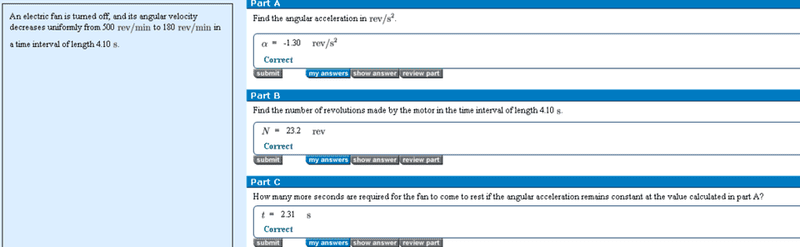# Angular motion with constant acceleration

[SOLVED] Angular motion with constant acceleration

## Homework Statement## Homework Equations

$$\varpi$$= $$\varpi$$(inital) + $$\alpha$$t

## The Attempt at a Solution

I understood part A and Part b,
What I don't understand is how to do Part C.
What I did was divide the average angular velocity with acceleration. But I don't get why or a more understanding method to do this problem

Hootenanny
Staff Emeritus
Gold Member
The angular acceleration is a measure of the rate of change of angular velocity, in other words, provided that the angular acceleration is constant it is the change in angular velocity divided by the time taken. Mathematically, for constant acceleration ($\alpha$),

$$\alpha = \frac{\Delta \omega}{\Delta t}$$

Which can be rearranged,

$$\Rightarrow \Delta t = \frac{\Delta \omega}{\alpha}$$

or,

$$\Delta t = \frac{\omega_\text{final} - \omega_\text{initial}}{\alpha}$$

In the case of part (c) you want to know how long it takes for the fan to come to rest, i.e. the final angular velocity is zero. Hence,

$$\Delta t = \frac{- \omega_\text{initial}}{\alpha}$$

Does that make sense?

Ahh that makes crystal clear perfect sense. Thank you so much Hootenanny!!